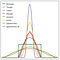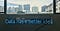# Mathematics behind Machine Learning: You need to become a better Data Scientist

It is not mandatory to understand the mathematics behind a model for you to make it work but it is crucial for success. No like you are using and experimenting with multiple black boxes and then using the one that work’s best.

So you can become a Data Scientist without the maths but you will heavily rely on luck and libraries 😓. To be successful, you need to understand the maths so that you can fine-tune your model but more importantly explain it with confidence 😎. …

# ML06: Intro to Multi-class Classification

This is a continuation of Mathematics behind Machine Learning Series.

In the last post, we saw how elegantly the maximum margin principle formulates to solve a binary classification task.

But for multiclass, the notion of maximum margin is harder to formulate. So the question of how can we reuse the 2-class formulation for k classes.

One-vs-Rest (OvR): for each class k train an SVM that is expert in classifying k (one) versus non-k (the rest) meaning we have to create a binary classifier for each class k, which is an expert in separating that class(k) from all the other classes.

# ML04: Kernel Density Estimation

This is a continuation of Mathematics behind Machine Learning Series.

Kernel density estimation is a non-parametric model also know as KDE, it’s a technique that lets you create a smooth curve given a set of data. KDE basically centers a kernel function at each data point and smooths it to get a destiny estimate.https://graphworkflow.com/eda/distributional-form/

The motivation behind the creation of KDM was that Histograms are not smooth, they depend on the width of the bins and the endpoints of the bins, KDMs reduce the problem by providing smoother curves.

# Investigating Customer Segmentation for Arvato Financial ServicesPhoto by Franki Chamaki on Unsplash

# Introduction

In this project, I have analyzed demographics data for customers of Bertelsmann Arvato Analytics in Germany, comparing it against demographics information for the general population and use that information and model to predict which individuals are most likely to convert into becoming customers.

Customer segmentation is the process of dividing customers into groups based on common characteristics so companies can market to each group effectively and appropriately.

# Problem statements

The analysis is divided into 3 major parts:

• Part 0: Get to Know the Data: I had a look at data and it’s structure and understood the data values. …

# This article will make Boston’s Airbnb hosts rethink their investment strategy.

## Based on analysis of Airbnb listing data in Boston

Airbnbs can inflow a sizable amount of earnings but owning this business is a difficult task. This article is for the owners to understand the market and make some simple but efficient decisions.

An important question a new host can ask is “How can I invest minimum but get higher rents”. The answer to that is simple- invest where it actually matters the most. In this blog, we will be using regression-based ML models to predict Airbnb prices and use the model to answer the above questions.

Let’s find answers to the following: